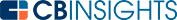# What are Depreciation Methods?A depreciation method is a means of calculating the overall amount of value goods will lose over their lifetime.

It’s often used to determine the amount of an asset’s value that a company can deduct on its taxes each year, although it can also be used to allocate the cost of an asset over its life expectancy. This enables companies to more accurately compare an asset’s expenses with the revenue it generates.

Depreciation is used to calculate how much value an asset loses at specific time intervals (typically a tax year) throughout the asset’s expected useful life. This accounting principle applies only to tangible assets. When referring to intangible assets such as loans or intellectual property, it’s known as amortization.

## Types of depreciation methods

Different types of depreciation methods offer different results and serve unique purposes for those who use them. The following methods are commonly used to calculate a variety of products' depreciation rates.

## The straight line method

This is arguably the most straight-forward method for calculating a product's depreciation rate.

To calculate a product’s annualized depreciation, you need to know its initial cost (the price it was purchased at or produced for), salvage value (the lowest acceptable value it can reach before being returned, sold, or recycled) and useful life (how long it can be expected to perform its intended function).

With these details in mind, you can calculate depreciation with the straight line method using the following formula:

Annualized depreciation cost = (product cost - salvage value) / useful life

What makes this method so simple to use is that the resulting depreciation cost is kept the same throughout the item's useful life until it eventually reaches its salvage cost.

## The double declining (or reducing) balance method

This method is fairly similar to the standard method mentioned above, but products or assets depreciate in value at twice the usual rate. The double declining balance method is often used to calculate the relative longevity of products that will soon be obsolete.

## The units of production method

In this method, the result is governed by the amount of use a given product or asset is projected to receive. This is often measured by the number of units it produces, hence the name.

## The sum of years' digits method

This method speeds up a given asset's expected depreciation rate early on in its existence, then slows this rate down as it ages. The idea behind this method is that the asset in question is likely to lose much of its value in its early years of functioning.

Depreciation methods are various ways to determine how much an asset loses its value over time. It’s used for spreading out the cost of an asset over time to align an asset’s expenses with the revenue it generates as well as for determining the portion of the cost that a company can deduct from its taxes each year throughout the asset’s useful lifespan.

You may also like# www.cbinsights.com